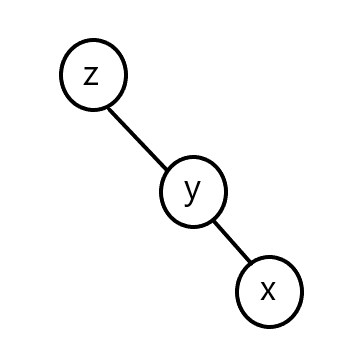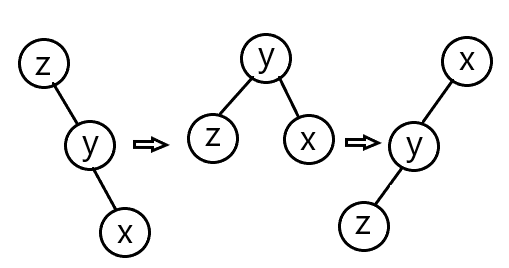# 前言

splay学了已经很久了，只不过一直没有总结，鸽了好久来写一篇总结。

# 算法思想

splay 是二叉搜索树的一种，这里简单介绍一下二叉搜索树。

splay 树在这上面进行了改进，通过不断改变树的形态来保证不会退化，均摊时间复杂度为 $$O(\log n)$$ 。基本思想是把搜索频率高的点放在深度小的位置，为了操作方便，可以认为每次操作的点都是频率高的。常常把操作的点，或是操作区间的两个端点放在根或根的附近的位置，那么会涉及到旋转操作。

# 基本操作

struct Splay_Node {
int son, val, cnt, siz, fa;
//分别是：两个儿子，权值，副本数，子树大小，父亲节点
#define ls t[pos].son //宏定义左儿子，方便一些
#define rs t[pos].son //右儿子，同上
};


## New

int New(int val, int fa) {
t[++tot].fa = fa, t[tot].cnt = t[tot].siz = 1, t[tot].val = val;
}


## Build

void Build() {
root = New(-INF, 0); //极小值为根节点
t[root].son = New(INF, root); //极大值为右儿子
}


## Ident

bool Ident(int pos) { return t[t[pos].fa].son == pos; }


## Update

void Update(int pos) {
t[pos].siz = t[ls].siz + t[rs].siz + t[pos].cnt; //子树大小为左右子树大小加上自己的副本数
}


## Connect

void Connect(int pos, int fa, int flag) {//依次是：子节点，父节点，哪个儿子
t[fa].son[flag] = pos;//将fa的儿子置为pos
t[pos].fa = fa;//将pos的父亲置为fa
}


## Rotate

### 左儿子旋转至父节点void Rotate(int pos) {//这里的flag1=0，可以按照上述的三个转换进行验证这段程序是对的
int fa = t[pos].fa, grand = t[fa].fa;
int flag1 = Ident(pos), flag2 = Ident(fa);
Connect(pos, grand, flag2);
Connect(t[pos].son[flag1 ^ 1], fa, flag1);
Connect(fa, pos, flag1 ^ 1);
Update(fa); Update(pos);
}


### 右儿子旋转至父节点

void Rotate(int pos) {//这里的flag1=1，可以按照上述的三个转换进行验证这段程序是对的
int fa = t[pos].fa, grand = t[fa].fa;
int flag1 = Ident(pos), flag2 = Ident(fa);
Connect(pos, grand, flag2);
Connect(t[pos].son[flag1 ^ 1], fa, flag1);
Connect(fa, pos, flag1 ^ 1);
Update(fa); Update(pos);
}


## Splay

### x、y、z 形成一条链### Code

void Splay(int pos, int to) {
for(int fa = t[pos].fa; t[pos].fa != to; Rotate(pos), fa = t[pos].fa)
if(t[fa].fa != to) Ident(pos) == Ident(fa) ? Rotate(fa) : Rotate(pos);
//Ident(pos) == Ident(fa)意味着pos和fa成为了一条链的形状，否则为“<”或“>”。
if(!to) root = pos;//更新根节点，根节点的父亲值为0
}


# 引申操作

## Find

int Find(int pos, int val) {
if(!pos) return 0;//空节点直接返回
if(val == t[pos].val) return pos;//等于就直接返回节点编号
else if(val < t[pos].val) return Find(ls, val);//在左子树中查找
else return Find(rs, val);//在右子树中查找
}


## Insert

1. 有权值为 $$val$$ 的点 $$pos$$ ，直接使得副本数加 $$1$$ 即可。
2. 没有权值为 $$val$$ 的点 $$pos$$ ，则开辟一个新的节点权值为 $$val$$

void Insert(int &pos, int val, int fa) {//pos为实参
if(!pos) Splay(pos = New(val, fa), 0);
else if(val == t[pos].val) { ++t[pos].cnt; Splay(pos, 0); }
else if(val < t[pos].val) Insert(ls, val, pos);
else Insert(rs, val, pos);
}


## Erase

1. 若当前节点的副本数大于 $$1$$ 时，即 $$t[pos].cnt>1$$ 时，可以删除其中一个副本即可，但并没有删除这个节点。
2. 否则，则需要删除该节点。需要先将 $$pos$$ splay 到根节点。找到它的前缀的编号 $$l$$ 和它的后缀的编号 $$r$$ ，则 $$t[l].val\leq val \leq t[r].val$$ 。显然， $$(t[l].val,t[r].val)$$ 区间内的数只有一个，即 $$pos$$ 。将 $$l$$ splay 至根节点， $$r$$ splay 至 $$l$$ 的右儿子，则 $$pos$$ 必会在 $$r$$ 的左儿子处，因为 $$l$$$$r$$$$pos$$ 必回满足二叉搜索树的性质。然后直接删除 $$r$$ 的左儿子即可。
void Erase(int val) {
int pos = Find(root, val);//找到权值为 val 的点。
if(!pos) return;//没有改节点直接返回，没有难倒删空气？
if(t[pos].cnt > 1) { --t[pos].cnt; Splay(pos, 0); return; }//对应情况1
Splay(pos, 0);
int l = ls, r = rs;
while(t[l].son) l = t[l].son;//找到前缀
while(t[r].son) r = t[r].son;//找到后缀
Splay(l, 0); Splay(r, l);//对应情况2
t[r].son = 0;
}


void Erase(int pos, int val) {
if(!pos) return;
if(val == t[pos].val) {
if(t[pos].cnt > 1) { t[pos].cnt--; Splay(pos, 0); return; }
if(ls) Rotate(ls), Erase(pos, val);//有左儿子跟左儿子交换
else if(rs) Rotate(rs), Erase(pos, val);//有右儿子就跟右儿子交换
else {//没有儿子就直接删除，注意必须删除其父亲的对应儿子
int newroot = t[pos].fa;
t[t[pos].fa].son[Ident(pos)] = 0;
Splay(newroot, 0);
}
return;
}
else if(val < t[pos].val) rase(ls, val);
else Erase(rs, val);
}


## Query_kth

1. $$val=t[pos].val$$ 时，即找到了该节点，返回比它小的数的个数即可，即左子树的节点数加 $$1$$
2. $$val<t[pos].val$$ 时， $$val$$ 左子树中，在左子树中查询该节点的排名。
3. $$val>t[pos].val$$ 时， 是最麻烦的部分。 $$val$$ 右子树中，左子树与当前节点都会为答案做贡献，先将其统计至答案中，在求出右子树对于答案的贡献。

int Query_kth(int pos, int val) {
if(!pos) return 0;//没有输出-1
if(val == t[pos].val) { int res = t[ls].siz + 1; Splay(pos, 0); return res; }//对应情况1
else if(val < t[pos].val) return Query_kth(ls, val);//对于情况2
//下两行代码对应情况3
int res = t[ls].siz + t[pos].cnt;//找到后splay维护形态会导致子树的大小变化，因此先记录答案
return Query_kth(rs, val) + res;
}


## Query_val

1. $$t[ls].siz\geq k$$ 时，即所求答案在左子树，在左边查询即可。
2. $$t[ls].siz+t[pos].cnt\geq k$$ 时， 答案为 $$t[pos].val$$ ，因为第 $$t[ls].siz+1$$ 小至 $$t[ls].siz+t[pos].cnt$$ 的数全部权值都为 $$t[pos].val$$
3. 否则，答案全部会在右子树当中，查询右子树第 $$k-t[ls].siz-t[pos].cnt$$ 大，因为当前节点与左儿子一定比右子树任何一个数小。

int Query_val(int pos, int rank) {
if(!pos) return INF;
if(t[ls].siz >= rank) return Query_val(ls, rank);
else if(t[ls].siz + t[pos].cnt >= rank) { Splay(pos, 0); return t[pos].val; }
return Query_val(rs, rank - t[ls].siz - t[pos].cnt);
}


## Get_Pre、Get_Nxt

$$Erase$$ 操作中提到过，可以使用那样的做法。

# C++代码

1. 插入 $$x$$ 数。
2. 删除 $$x$$ 数(若有多个相同的数，因只删除一个)。
3. 查询 $$x$$ 数的排名(排名定义为比当前数小的数的个数 $$+1$$ )。
4. 查询排名为 $$x$$ 的数。
5. $$x$$ 的前驱(前驱定义为小于 $$x$$，且最大的数)。
6. $$x$$ 的后继(后继定义为大于 $$x$$，且最小的数)。

#include <cstdio>
namespace Quick_Function {
template <typename Temp> void Read(Temp &x) {
x = 0; char ch = getchar(); bool op = 0;
while(ch < '0' || ch > '9') { if(ch == '-') op = 1; ch = getchar(); }
while(ch >= '0' && ch <= '9') { x = (x << 1) + (x << 3) + (ch ^ 48); ch = getchar(); }
if(op) x = -x;
}
template <typename Temp> Temp Max(Temp x, Temp y) { return x > y ? x : y; }
template <typename Temp> Temp Min(Temp x, Temp y) { return x < y ? x : y; }
template <typename Temp> Temp Abs(Temp x) { return x < 0 ? (-x) : x; }
template <typename Temp> void Swap(Temp &x, Temp &y) { x ^= y ^= x ^= y; }
}
using namespace Quick_Function;
#define INF 0x3f3f3f3f
const int MAXN = 1e6 + 5;
int n;
struct Splay_Node {
int son, val, cnt, siz, fa;
#define ls t[pos].son
#define rs t[pos].son
};
struct Splay_Tree {
int root, tot;
Splay_Node t[MAXN];
bool Ident(int pos) { return t[t[pos].fa].son == pos; }
int New(int val, int fa) {
t[++tot].fa = fa, t[tot].cnt = t[tot].siz = 1, t[tot].val = val;
}
void Build() { root = New(-INF, 0); t[root].son = New(INF, root); }
void Update(int pos) { t[pos].siz = t[ls].siz + t[rs].siz + t[pos].cnt; }
void Connect(int pos, int fa, int flag) { t[fa].son[flag] = pos, t[pos].fa = fa; }
void Rotate(int pos) {
int fa = t[pos].fa, grand = t[fa].fa;
int flag1 = Ident(pos), flag2 = Ident(fa);
Connect(pos, grand, flag2);
Connect(t[pos].son[flag1 ^ 1], fa, flag1);
Connect(fa, pos, flag1 ^ 1);
Update(fa); Update(pos);
}
void Splay(int pos, int to) {
for(int fa = t[pos].fa; t[pos].fa != to; Rotate(pos), fa = t[pos].fa)
if(t[fa].fa != to) Ident(pos) == Ident(fa) ? Rotate(fa) : Rotate(pos);
if(!to) root = pos;
}
int Find(int pos, int val) {
if(!pos) return 0;
if(val == t[pos].val) return pos;
else if(val < t[pos].val) return Find(ls, val);
else return Find(rs, val);
}
void Insert(int &pos, int val, int fa) {
if(!pos) Splay(pos = New(val, fa), 0);
else if(val == t[pos].val) { ++t[pos].cnt; Splay(pos, 0); }
else if(val < t[pos].val) Insert(ls, val, pos);
else Insert(rs, val, pos);
}
void Erase(int val) {
int pos = Find(root, val);
if(!pos) return;
if(t[pos].cnt > 1) { --t[pos].cnt; Splay(pos, 0); return; }
Splay(pos, 0);
int l = ls, r = rs;
while(t[l].son) l = t[l].son;
while(t[r].son) r = t[r].son;
Splay(l, 0); Splay(r, l);
t[r].son = 0;
}
int Query_kth(int pos, int val) {
if(!pos) return 0;
if(val == t[pos].val) { int res = t[ls].siz + 1; Splay(pos, 0); return res; }
else if(val < t[pos].val) return Query_kth(ls, val);
int res = t[ls].siz + t[pos].cnt;
return Query_kth(rs, val) + res;
}
int Query_val(int pos, int rank) {
if(!pos) return INF;
if(t[ls].siz >= rank) return Query_val(ls, rank);
else if(t[ls].siz + t[pos].cnt >= rank) { Splay(pos, 0); return t[pos].val; }
return Query_val(rs, rank - t[ls].siz - t[pos].cnt);
}
int Get_Pre(int val) {
int pos, res, newroot;
pos = newroot = root;
while(pos) {
if(t[pos].val < val) { res = t[pos].val; pos = rs; }
else pos = ls;
}
Splay(newroot, 0);
return res;
}
int Get_Nxt(int val) {
int pos, res, newroot;
pos = newroot = root;
while(pos) {
if(t[pos].val > val) { res = t[pos].val; pos = ls; }
else pos = rs;
}
Splay(newroot, 0);
return res;
}
};
Splay_Tree tree;
int main() {
for(int i = 1, opt, x; i <= n; i++) {